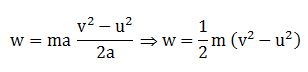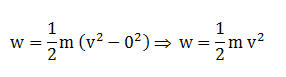# Derivation of the equation for kinetic energy (K.E = ½ m v 2)

Derivation of the equation for kinetic energy (K.E = ½ m v 2)

From equations of motion, the relation connecting the initial velocity (u) and final velocity (v) of an object moving with a uniform acceleration a, and the displacement, s is
But from Newton’s second law of motion F = m a.
work done by the force, F is written as the following:If the object is starting from its stationary position, that is, u = 0, thenFrom work and energy theorem, work done is equal to the change in the kinetic energy of the object.
w = (1/2) mv 2
If u = 0, the work done will be,
Thus the kinetic energy possessed by an object of mass, m and moving with a uniform velocity, v is
K.E = (1/2) mv 2